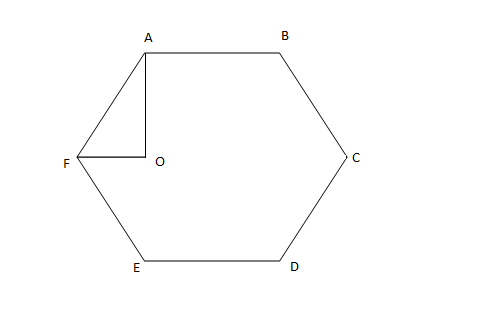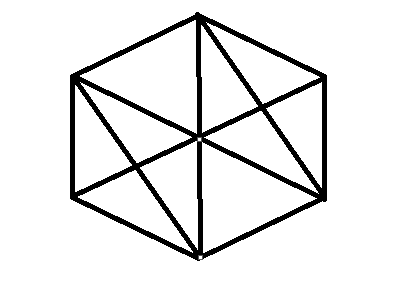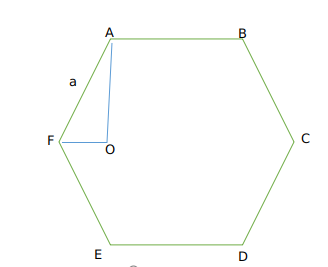Question 80

# In the figure below, ABCDEF is a regular hexagon and $$\angle{AOF}$$ = 90° . FO is parallel to ED. What is the ratio of the area of the triangle AOF to that of the hexagon ABCDEF?SolutionWhen the hexagon is divided into number of similar triangle AOF we get 12 such triangles . Hence required ratio of area is 1/12.
Alternate Approach :Let us take side of the hexagon as a
Now We get AF = a
Now As OF || ED
Angle OFE +Angle E = 180
We know Angle E =120
So angle OFE=60 degrees.
Now therefore Angle AFO=120-60=60 degrees
Now in triangle AFO
Cos60=$$\frac{OF}{AF}$$
$$\frac{1}{2}=\frac{OF}{AF}$$
We get OF = $$\frac{a}{2}$$
Now therefore area of triangle AOF =$$\frac{1}{2}\times\ AF\times\ OF\times\ \sin60$$
So we get $$\frac{1}{2}\times\ a\times\ \frac{a}{2}\times\ \frac{\sqrt{\ 3}}{2}$$
=$$\frac{\sqrt{\ 3}}{8}a^2$$     (1)
Now area of hexagon = $$6\times\ \frac{\sqrt{\ 3}}{4}a^2$$      (2)
Dividing (1) and (2) we get ratio as $$\frac{1}{12}$$
Hence option A is the correct answer.